### 13.4.1.2 Hamilton's principle of least action

Now sufficient background has been given to return to the dynamics of mechanical systems. The path through the C-space of a system of bodies can be expressed as the solution to a calculus of variations problem that optimizes the difference between kinetic and potential energy. The calculus of variations principles generalize to any coordinate neighborhood of. In this case, the Euler-Lagrange equation is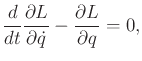(13.124)

in which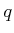is a vector ofcoordinates. It is actuallyscalar equations of the form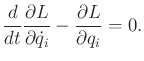(13.125)

The coming presentation will use (13.124) to obtain a phase transition equation. This will be derived by optimizing a functional defined as the change in kinetic and potential energy. Kinetic energy for particles and rigid bodies was defined in Section 13.3.1. In general, the kinetic energy function must be a quadratic function of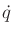. Its definition can be interpreted as an inner product on, which causesto become a Riemannian manifold . This gives the manifold a notion of the angle'' between velocity vectors and leads to well-defined notions of curvature and shortest paths called geodesics. Let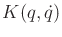denote the kinetic energy, expressed using the manifold coordinates, which always takes the form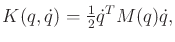(13.126)

in which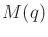is an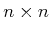matrix called the mass matrix or inertia matrix.

The next step is to define potential energy. A system is called conservative if the forces acting on a point depend only on the point's location, and the work done by the force along a path depends only on the endpoints of the path. The total energy is conserved under the motion of a conservative system. In this case, there exists a potential function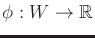such that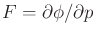, for any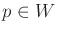. Let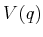denote the total potential energy of a collection of bodies, placed at configuration.

It will be assumed that the dynamics are time-invariant. Hamilton's principle of least action states that the trajectory,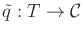, of a mechanical system coincides with extremals of the functional,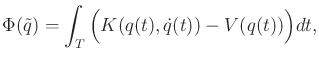(13.127)

using any coordinate neighborhood of. The principle can be seen for the case of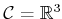by expressing Newton's second law in a way that looks like (13.124) :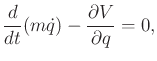(13.128)

in which the force is replaced by the derivative of potential energy. This suggests applying the Euler-Lagrange equation to the functional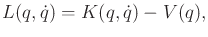(13.129)

in which it has been assumed that the dynamics are time-invariant; hence,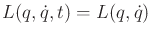. Applying the Euler-Lagrange equation to (13.127) yields the extremals.

The advantage of the Lagrangian formulation is that the C-space does not have to be, described in an inertial frame. The Euler-Lagrange equation gives a necessary condition for the motions in any C-space of a mechanical system. The conditions can be expressed in terms of any coordinate neighborhood, as opposed to orthogonal coordinate systems, which are required by the Newton-Euler formulation. In mechanics literature, thevariables are often referred to as generalized coordinates. This simply means the coordinates given by any coordinate neighborhood of a smooth manifold.

Thus, the special form of (13.124) that uses (13.129) yields the appropriate constraints on the motion: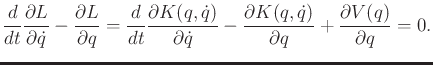(13.130)

Recall that this representsequations, one for each coordinate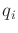. Sincedoes not depend on time, the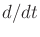operator simply replacesby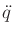in the calculated expression for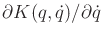. The appearance ofseems appropriate because the resulting differential equations are second-order, which is consistent with Newton-Euler mechanics.

Example 13..11 (A Falling Particle)   Suppose that a particle with mass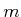is falling in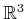. Let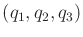denote the position of the particle. Let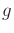denote the acceleration constant of gravity in the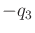direction. The potential energy is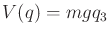. The kinetic energy is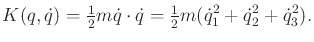(13.131)

The Lagrangian is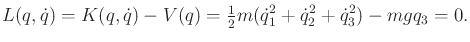(13.132)

To obtain the differential constraints on the motion of the particle, use (13.130). For eachfromto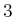,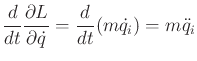(13.133)

Sincedoes not depend on, the derivative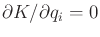for each. The derivatives with respect to potential energy are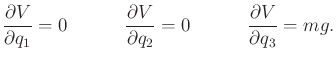(13.134)

Substitution into (13.130) yields three equations: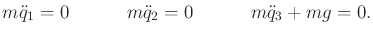(13.135)

These indicate that acceleration only occurs in thedirection, and this is due to gravity. The equations are consistent with Newton's laws. As usual, a six-dimensional phase space can be defined to obtain first-order differential constraints.The least'' part of Hamilton's principle is actually a misnomer. It is technically only a principle of extremal'' action because (13.130) can also yield motions that maximize the functional.

Steven M LaValle 2020-08-14